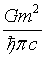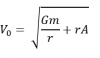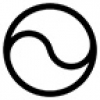## Hypersphere CosmologyAlternative to big-bang

Thursday, 04 December 2014 09:38

## Hypersphere Holometry - A PredictionAs a consequence of the hypothesis that the universe exists as a hypersphere wherethe main parameters of the universe all come out at the same multiple of the corresponding Planck quantities:Where U stands for a very large dimensionless number now called the Ubiquity Constant.

Now the Beckenstein-Hawking conjecture about black hole entropy initiated speculation about the idea of a ‘holographic universe’ because if we equate entropy with information then it suggests that the amount of information in the universe corresponds to its surface area and perhaps the information somehow lies on its surface and the apparent 3d universe just consists of a projection from that. Personally I don’t buy into that hypothesis but I do think the basic conjecture tells us something about reality because the universe does not appear to contain enough information to allow for quantisation right down to the Planck scales.

Various experiments are in progress to see if the universe actually exhibits spacetime quantisation, see http://en.wikipedia.org/wiki/Holometer for links.

Now the Beckenstein-Hawking conjecture states:

I =Where I = information in bits if we equate information with entropy, (eliminating Boltzmann’s constant in the process). A = the surface area of the universe, and here we can use either the ‘causal horizon’ from the big bang or the spatial horizon from the hypersphere cosmology hypothesis.means four times the Planck length squared.

So a calculation can proceed as follows: -

Calculating the surface area of universe A, and then dividing byA = 4πr =A =Dividing area by 4Area/4=where ђ means Planck’s constant reduced.

This comes out at2.0559 x, the number of bits for the universe, the number of bits for the universe.

Calculating the number of Planck volumes in the universe:

Volume of universe == 3.314 xCalculating the number of Planck volumes in the universe per bit in the universe:

3.314 x 10183 / 2.0559 x 10120 = 160 x 1060

Taking the cube root of this for the number of bits per Planck length = 5.4 x1020

Thus the model predicts that:

*The universe will exhibit an effective ‘pixilation’ or ‘grain size’ or quantisation of spacetime at Planck length and Planck time x 5.4 x 1020

Note that a derivation using the same Beckenstein-Hawking formula for a 13.8 bnlyr universe will give a different prediction because this model usesfor hypersphere volume rather thanc

*Note also that we have yet to observe anything ‘real’ below these new limits.

Plus this may have interesting implications for the Heisenberg uncertainty relationships.

• #### HyperSphere Cosmology +Hypersphere Cosmology in a Nutshell   1a) The Universe consists of a Hypersphere (a 3-Sphere or 4-ball) where: -       also
• #### Hypersphere Redshift +The alternative mechanism for redshift works as follows: Redshift = Z =   - 1 Where = observed wavelength, = expected wavelength, and the -1
• #### Hypersphere Rotation +The Gödel 3d rotating universe has this solution derived from General Relativity: - “Matter everywhere rotates relative to the compass of
• #### Hypersphere Holometry - A Prediction +As a consequence of the hypothesis that the universe exists as a hypersphere where   the main parameters of the
• #### VHC. Vorticitating Hypersphere Cosmology +The overwhelming majority of university and state funded cosmologists believe that the observable universe has expanded from a much smaller
• #### Hypersphere Visualisation & Lensing +This paper provides a method of visualising the four dimensional hypersphere as a perspective construction in three dimensions, and it
• #### Sacred Geometry +

As faith began to make way for reason during the Enlightenment, humans began to deduce the relationships between certain mathematical
• #### Starships +

Houston we have a problem. If the human race does not develop starships that can reach other star systems as
• #### Quantum & Probability +

I have repeatedly asserted in many of my magical and scientific books and papers that this universe runs on probability
• #### Ur Chaos Theory +

In this paper 'Chaos' denotes randomness and indeterminacy, not the mere so-called 'deterministic chaos' of systems with extreme sensitivity to
• 1